Half Life Time, Mean Life & Radioactivity Series

# Half Life Time, Mean Life & Radioactivity Series - Inorganic Chemistry

16. Half-life (T1/2):

(a) The time in which the number of atoms (N) reduces to half of its initial value (N0) is defined as the halflife of the element (i.e. half of the atoms decay).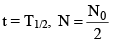(b) The time in which the activity reduces to half of its initial value is defined as half life.

At t = T1/2,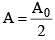(c) Its unit is second
(d) Formulae of half life

(i)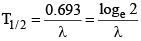(ii)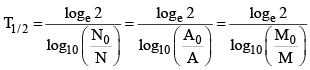(iii) T1/2 = 1/n where n = No. of half life

(iv) Time of disintegration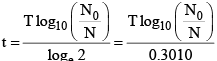17. Mean life (t):

(a) The time, for which a radioactive material remains active, is defined as mean life of that material:

(b)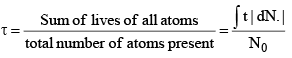(c) The average time taken in decaying by the atoms of an element is defined as its mean life t.
(d) τ = 1/λ
(e) Its units are second, minute, hour day, month, year etc.
(f) Mean life does not depend on the mass of material. It depends on the nature of the material.
(g) The magnitude of slope of decay curve is equal to the mean life.
(h) Relation between the mean life and half -life.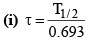(ii) τ = 1.44 T1/2
(iii) τ > T1/2

(iv) The time, in which the number of radioactive atoms decays to 1/e or 37% of its initial value, is defined as the mean life of that material.

18. Important Formulae Related to Law of Disintegration (τ):

(a) N = N0e–λt
(b) A = A0e–λt
(c) M = M0e-λt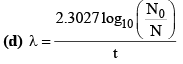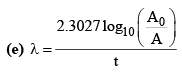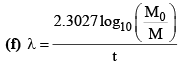(g) λ = λα + λβ

(h)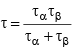(where two particles decay simultaneously)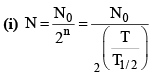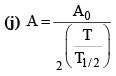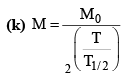19. Useful Hints:

(i) Percentage decreases in activity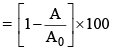(ii) Number of atoms remaining after n half lives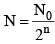(iii) Number of atoms decayed after time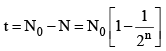(iv) The fraction of radioactive material at time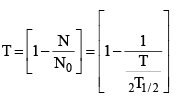(v) Percentage of radioactive material decayed at time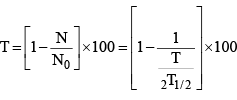(vi) Percentage of radioactive material decayed in n half lives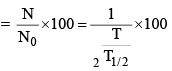(vii) Fraction of radioactive material decayed in n half lives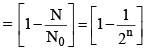(viii) Percentage of radioactive material decayed in n half lives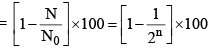(ix) Percentage of radioactive material remaining after n half-lives.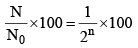(x) When decay process is too slow then N = N0 – N0λt or N = - (N0λ)t + N0

(xi) N-t graph is a straight line with -ve slope, for slow decay process.

20. Characteristics of α, β and γ rays

 S. No. Property α-Particles β-Particles γ-rays 1. Natural           andvalue of charge Positive and double of the charge of the proton Negative and equal to the charge of electron 1.6 × 10-19 C Uncharged(Nautral) 2. Nature              ofparticle Doubly ionized helium atom (2 protons and 2 neutrons) Electron (or) position Electromagneticwaves 3. Mass Four times the mass of the proton (4 × 1.67 x 10-27 kg) Equal to the mass of electron 9.1 x 10-31 kg Mass less
 4 Specific charge q/m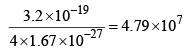1.7 × 1011 Ckg–1 Uncharged and mass less 5 Explained by Tunnel effect Neutrino hypothesis Transitions         ofnuclei into the ground        energylevel after a and P-decay 6 Effect of electric and        magneticfields Deflected by electric and magnetic fields Deflected by electric and magnetic fields Unaffected 7 Penetratingpower 1 100 10000 8 Ionizing power 100000 100 1 9 Velocity Less than the velocity of light (1.4 × 104 m/s to 2.2 x 107 ms-1 Approximately equal to the velocity of light 3 × 108 m/s 10 Mutualinteraction with matter Produce heat Produce heat Produce            thephenomenon of Photoelectric effect, Compton

21. α-emission

(a) Characterstictics of α-decay:

(i) The spectrum of α-particles is a discrete line spectrum.
(ii) Spectrum of α-particles has fine structure i.e. every spectral line consists of a number of fine lines.
(iii) The α-emitt ing nuclei have discrete energy levels i.e energy levels in nuclei are analogous to discrete energy levels in atoms.
(iv) α-decay is explained on the basis of tunnel effect.

(v) Geiger-Muller law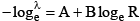for radioactive series B is same whereas A is different.

(b) Range of α-particles:

(i) The maximum distance traversed by α-particles in air before being finally stopped is defined as the range of α-particles.
(ii) The maximum distance traversed by α-part icles before being finally absorbed after io nizing gas molecules, is defined as the range of α-particles.
(iii) The range of α-part icles in air is fro m 2.6 cm to 8.6 cm.
(iv) Relations between the range of α-particles and their energy

(I) R = 0.318 E3/2
(II) log R = log 0.318 + 3/2 log E
(c) Size of the nucleus decreases by α-emission

22. Characteristics of β-decay:

(i) The energy spectrum of β-particles is continuous i.e. β-particles of all energies upto a certain maximum are emitted.
(ii) The number of such β-particles is maximum whose energy is equal to the maximum probable energy i.e. at E = Emp, NB = maximum
(iii) There is a characteristic maximum value of energy in the spectrum of β-particles which is known as the end point energy (E0)
(iv) In β-decay process, a neutron is converted into proton or proton is converted into neutron.

0n1 = 1p1 + –1e0 (β– Particle)

1p1 = 0n1 + 1e0+ Particle)

(v) The energy of β-particles emitted by the same radioactive material may be same or different.

(vi) The energy of β-particles with energy E = E0 (end point energy) is zero.

23. Neutrino Hypothesis:

(a) According to Pauli, whenever neutron is converted into proton or proton into neutron then this process is accompanied with the emission of a new particle to which he named as neutrino.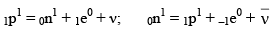(b) Properties of neutron:
(i) The charge on neutrino is zero
(ii) The rest mass of neutrino is zero

(iii) Its spin angular momentum is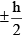(iv) Its speed is equal to that of light
(v) It has finite magnetic moment but the magnitude is very small
(vi) It antiparticle is ant i-neutrino.

(vii) The linear momentum vector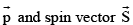are mutually in opposite directions.

(viii) Its energy is equal to (Eend – Eβ)

(ix) It does not interact with matter.

(x) Neutrino was discovered by Pauli and its experimental verificat ion is done by Reines and Cowan.

24. Characterstics of γ-decay

(i) The spectrum of γ-rays is a discrete line spectrum
(ii) Whenever, α or β-particles is emitted by a nucleus then the daughter nucleus is left in the excited state. It suddenly makes transition in the ground state thereby emitting γ-rays. (iii) Knowledge about nuclear energy levels is obtained by γ-spectrum.
(iv) γ-rays interact with matter as a consequence of which the pheno mena of photoelectric effect, Compton effect and pair production happen. (At low energy photoelectric effect and at high energy pair-production are effective).

25.  Intensity of γ-rays in materials

(i) When γ-rays penetrate matter, then their intensity (a) decreases exponentially with depth (x) inside the matter. The intensity of γ-rays at depth x inside the matter is given by I = I0e–μx
(ii) The thickness of matter, at which the intensity of γ-rays (I) reduces to half its initial maximum value (I0), is known as its half-life value thickness.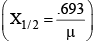(iii) The reciprocal of the distance inside matter, at which the intensity (I) reduces to 1/e

or 37% of its maximum value (I0), is defined as the coefficient of absorption (μ) of that material.

(iv) Coefficient of absorption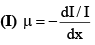(II) μ depends on the wavelength of γ-rays (μ α λ3) and the nature of absorbind material.

If parent element is unstable then it will dissociate into daughter element & if this daughter element is still unstable, then it will again dissociate into a new daughter element & process continuous till the format ion of a stable element. Series of element obtained fro m parent element to the finally stable non-radioactive element is known as radioactive disintegration series.

(4n +1) is artificial series & (4n + 2), (4n +3) are natural series.

 S. No. Series Name of the series Initialelement Finalelement Nature of series No of α & βParticlesemitted 1. 4n + 2 Uranium series 92U238 82Pb206 Natural 8α, 6β 2. 4n + 3 Actinium series 92U235 82Pb207 Natural 7α, 4β 3. 4n Thorium series 90Th232 82Pb208 Natural 6α, 4β 4. 4n + 1 Neptunium series 93U237 83Bi209 Artificial 7α, 4β

27. To Calculate no of α-particles and β-Particles emitted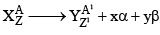x: no of α-particles emitted y: no of β-particles emitted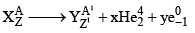A = A1 + 4x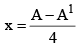Z = Z1 + 2x – y

y = Z1 – Z + 2x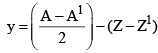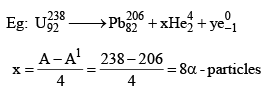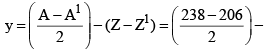(92 - 82) = 16 - 10 = 6β - particles

The unit of radioactivity is curie (Ci). It is the quantity of any radioactivity substance which has decay rate of 3.7 × 1010 disintegrat ions per second.

1 millicurie (mCi) = 3.7 × 107 disintegrat ions per sec.

1 microcurie (μCi) = 3.7 × 104 disintegrat ions per sec.

There is another unit called Rutherford (Rd) which is defined as the amount of a radioactive substance which undergoes 106 disintegrat ions per second.

1 milli Rutherford = 103 disintegrat ion per sec.

1 micro Rutherford = 1 disintegration per sec.

The SI unit radioactivity is proposed as Becquerel which refers to one dps.

1 curie = 3.7 × 104 Rutherford.

1 curie = 3.7 GBq

Here, G stands for 109, i.e., giga.

29. Isotopes, Isobars and Isotones:

 S. No. Isotopes Isobars Isotones 1. The atoms of the same elements whose charge number (Z) is same but mass number is different are known as isotopes. The atoms with mass number same and charge numberdifferent are known as isobars. The atoms with same neutron number but A and Z are different are known as isotones 2. Chemical properties are same Chemical properties are different Chemical properties are different 3. Number of electrons is same Number of electrons is different Number of properties are different 4. Occupy same place in periodic table Occupy differentplaces in periodic table Occupy differentplaces in periodic table. 5. Example:8O16, 8O17, 8O18, 1H1, 1H2, 1H3, 10Ne20, 10Ne20, 1oNe21, 1oNe22 1H3 and 2He36C14 and 7N14 8O17 and 9F17 3Li7 and 4Be81H2 and 2He31H3 and 2He4

They are two types.

(a) Natural radioactive isotopes: Those radioact ive isotopes which exist naturally are known as natural radioactive isotopes. e.g.Th232, Pu240 etc.

(b) Artificial radioactive isotopes: Those isotopes, which are prepared artificially by bo mbarding fundamental particles like α, β, γ, p, n etc, no matter, are known as artificial isotopes.

The document Half Life Time, Mean Life & Radioactivity Series | Inorganic Chemistry is a part of the Chemistry Course Inorganic Chemistry.
All you need of Chemistry at this link: Chemistry

## Inorganic Chemistry

40 videos|90 docs|41 tests

## FAQs on Half Life Time, Mean Life & Radioactivity Series - Inorganic Chemistry

 1. What is half-life time and how is it calculated?Ans. Half-life time is the time taken for the radioactivity of a substance to decrease by half. It is calculated using the equation T1/2 = (0.693 / λ), where T1/2 is the half-life time and λ is the decay constant.
 2. What is the mean life of a radioactive substance?Ans. The mean life of a radioactive substance is the average time taken for the radioactivity of a substance to decrease by half. It is calculated using the equation τ = 1 / λ, where τ is the mean life and λ is the decay constant.
 3. What is a radioactivity series?Ans. A radioactivity series is a sequence of radioactive isotopes that are formed as a result of the decay of a parent radioactive isotope. Each isotope in the series undergoes a specific decay process, leading to the formation of another isotope in the series.
 4. How do half-life time and mean life relate to each other?Ans. Half-life time and mean life are related to each other through the equation T1/2 = 0.693 * τ, where T1/2 is the half-life time and τ is the mean life. This equation shows that the half-life time is approximately 0.693 times the mean life.
 5. Can the half-life time or mean life of a radioactive substance be altered?Ans. No, the half-life time and mean life of a radioactive substance are intrinsic properties that are determined by the nature of the radioactive isotope. They cannot be altered or influenced by external factors such as temperature, pressure, or chemical reactions.

## Inorganic Chemistry

40 videos|90 docs|41 testsExplore Courses for Chemistry examSignup to see your scores go up within 7 days! Learn & Practice with 1000+ FREE Notes, Videos & Tests.
10M+ students study on EduRev
Track your progress, build streaks, highlight & save important lessons and more!
Related Searches

,

,

,

,

,

,

,

,

,

,

,

,

,

,

,

,

,

,

,

,

,

,

,

,

;